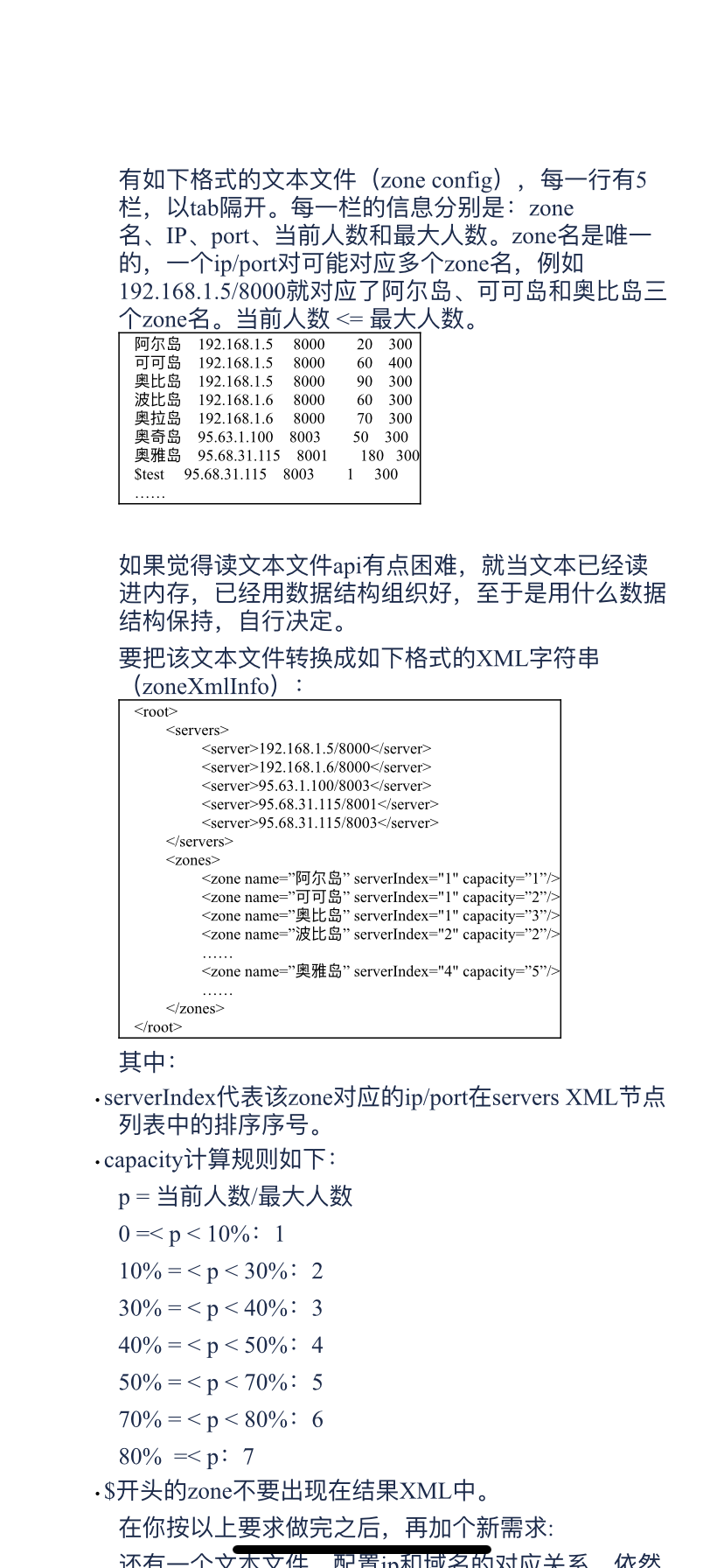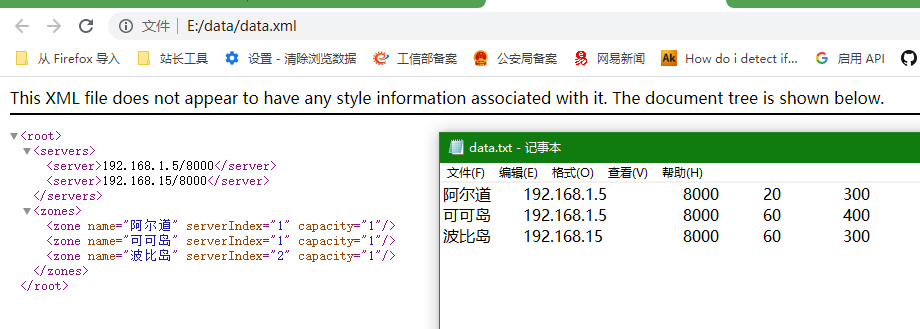2021-07-20 10:50

# c#解决txt文档写成xml• 收藏

#### 2条回答默认 最新

•CSDN专家-showbo 2021-07-20 11:01
已采纳

有帮助麻烦点个采纳【本回答右上角】，谢谢~~ 注意修改文件路径和编码``````using System;
using System.Text;
using System.IO;
using System.Collections.Generic;
namespace ConsoleApplication1
{
class Program
{
static void Main(string[] args)
{
//注意修改这里的text路径，如果文件存储编码不是utf-8，也要修改编码

StringBuilder sb = new StringBuilder("<zones>");
Dictionary<string, string> server = new Dictionary<string, string> { };
string key;
int index = 1;
foreach (var item in items)
{
if (item.Trim() == "" || item.StartsWith("\$")) continue;//空项或者\$开头继续
var arr = item.Split('\t');//拆分

key = arr + '/' + arr;//server
if (!server.ContainsKey(key))//新server加入
{
index++;
}
var p = double.Parse(arr) / double.Parse(arr);
string capacity = "";
if (p >= 0 && p < 10) capacity = "1";
else if (p >= 10 && p < 30) capacity = "2";
else if (p >= 30 && p < 40) capacity = "3";
else if (p >= 40 && p < 50) capacity = "4";
else if (p >= 50 && p < 70) capacity = "5";
else if (p >= 70 && p < 80) capacity = "6";
else capacity = "7";

sb.Append("<zone name=\"" + arr + "\" serverIndex=\"" + server[key] + "\" capacity=\"" + capacity + "\"/>");

}
sb.Append("</zones>");

string sserver = "<servers>";
foreach (var k in server.Keys) sserver += "<server>" + k + "</server>";
sserver += "</servers>";

Console.WriteLine("<root>"+ sserver + sb.ToString()+"</root>");//这个就是你要的xml格式字符串
//要写入文件，注意修改路径
File.WriteAllText(@"E:\data\data.xml", "<root>" + sserver + sb.ToString() + "</root>", Encoding.UTF8);

}
}
}
``````
打赏 评论
•li.siyuan 2021-07-20 11:22

请详细描述你的问题,
txt 文件的内容 示例
xml 文件转换后的内容 示例

请采纳

打赏 评论